# Manifold

A geometric object which locally has the structure (topological, smooth, homological, etc.) ofor some other vector space. This fundamental idea in mathematics refines and generalizes, to an arbitrary dimension, the notions of a line and a surface. The introduction of this idea was influenced by various requirements, both of mathematics itself and of other sciences. In mathematics, manifolds arose first of all as sets of solutions of non-degenerate systems of equations and also as various sets of geometric and other objects allowing local parametrization (see below); for example, the set of planes of dimensionin. They also appear as solutions of multi-dimensional variational problems (soap films), as integral manifolds of Pfaffian systems and of dynamical systems, as groups of geometric transformations and their homogeneous spaces, etc. In physics they play the role of models of space-time, in mechanics they act as phase spaces, energy levels, etc., in economics as surfaces of indifference, in psychology as spaces of sensations (for example, colours), etc.

Although the initial idea underlying the definition of a manifold is that of a local structure ( "the very same as Rn" ), this idea admits a whole series of global features typical for manifolds: (non-) orientability, homological Poincaré duality, the possibility of defining the degree of a mapping of one manifold onto another of the same dimension, etc. Of particular significance is the introduction of the tangent bundle and its related invariants.

The local structure of a manifold also allows the use of geometric techniques: putting into general position, the construction of Morse functions (cf. Morse function), etc., which are used for the geometric study of the global structure of manifolds, and, roughly speaking, consist of constructing a possibly simpler image of a manifold as a union of simple pieces; for instance, simplices or handles.

To make use of the idea of a manifold a transition from the local to the global point of view is usually made. The first stage is the introduction of a parametrization, that is, a representation of the "state space" of a given problem as a domain in a space of numbers. This gives one the possibility to describe by a set of numbers the coordinates of the corresponding point (the coordinate method). In the large the state space need not admit a similar description, that is, it need not be homeomorphic to a region in. If one does not resort to parametrizations with degeneracies (as in polar coordinates and their generalizations), then two paths are possible: either to introduce from the outset a larger than necessary number of parameters, and to find then the actual space implicitly using a system of equations ( "state equations" ), or to parametrize the space locally ( "in the small" ). For example, the set of lines in a plane is covered by two subsets:, consisting of lines with equations of the form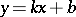, and, consisting of lines with equations of the form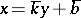; both of these are homeomorphic to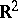with parametrizations by the pairsand, respectively. However, in the large, this set is homeomorphic to an open Möbius strip, and hence not like.

When manifolds appear naturally in some domain or other, they are sure to carry some additional structure, which also is a topic of study in the domain. However, a major role is played by the topological structure, restricting the a priori possibilities. Conversely, in topology, the local and global properties of manifolds are studied taking into consideration the extra structures (for example, smooth) as tools.

The basis of the general idea of a manifold is the definition of a topological manifold as a topological space in which each point has a neighbourhoodand a homeomorphism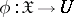onto a domain inor into the half-space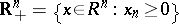; the homeomorphismis called a local parametrization, or chart, in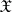. For a connected manifold, the dimension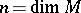is an invariant. For a disconnected manifold the components are usually taken to be of the same dimension. A manifold decomposes into an interiorand a boundary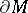(also called edge): points of the boundary correspond in the charts to the points of the boundary ofin. The boundary is an-dimensional manifold without boundary and need not be empty. A connected manifold without boundary is called open if it is non-compact, and closed if it is compact. The simplest examples of the four possible types of manifold are,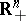, the ball, and its boundary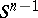. Although non-Hausdorff manifolds occur in certain situations (for example, the total space of a sheaf), it is usually assumed that a manifold is Hausdorff, paracompact, has a countable base, and, in particular, is metrizable.

The global specification of a manifold is accomplished by an atlas: A set of charts covering the manifold. To use manifolds in mathematical analysis it is necessary that the coordinate transitions from one chart to another are differentiable. Therefore differentiable manifolds (cf. Differentiable manifold) are most often considered. A more general form is introduced by the idea of a-structure on a manifold, given by atlases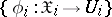, in which the coordinate transitions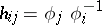are homeomorphisms in a systemof mappings of domains inthat is closed relative to composition. Ifconsists oftimes continuously-differentiable mappings, then one says that the smoothness class of the manifold is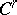. In a similar way one defines analytic manifolds (cf. Analytic manifold), and piecewise-linear, Lipschitz, etc., types of manifolds. Two-atlases give a single-structure if their union is a-atlas. The classification of-structures is the most important problem in the geometry of manifolds. A mappingof one-manifold into another is called a-mapping if locally it has a "coordinate representation", whereare charts inandand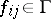. In particular, there is the notion of a-homeomorphism (a-diffeomorphism if).

Since, in mathematical analysis, manifolds are important as carriers of differentiable mappings, they are sometimes defined (see ) by their set of smooth functions, defined in neighbourhoods of points (see Sheaf). The development of this idea has led to the idea of a pre-manifold or a ringed space (a sheaf of rings over a space), and further to the notion of a scheme. Replacingby vector or other spaces, one arrives at various generalizations of a manifold such as, for example, complex-analytic manifolds. Infinite-dimensional manifolds arose in mathematical analysis and topology as spaces of mappings and sections of bundles, as spaces of homeomorphisms, spaces of closed subsets, etc. Their local models are vector spaces (Banach spaces, etc.) and spaces such as the Hilbert cube. The notions of smooth and other structures on infinite-dimensional spaces have not been sufficiently studied. A difficulty arises here because of the absence of technical theorems on approximations, existence of partitions of unity (a stock of small smooth functions), implicit functions, etc.

Manifolds being implicitly given as sets of solutions of systems of equations (inequalities in the case of a non-empty boundary) arise as subsets of. These manifolds are given all at once, and not in pieces, as with the assignment of an atlas. However, a necessary condition is non-degeneracy, otherwise every closed set can be given by one equation. The existence of a local parametrization is provided by the implicit-function theorem from the condition of maximal rank of the Jacobi matrix of the system. The equations are used as a language for expressing, by mathematical analysis, the properties of a manifold and are used to define the manifold. For example, the property of orthogonality of-matrices is given by a system ofequations relative to the entries of the matrix. The system turns out to be non-degenerate, and the group of orthogonal matrices is a smooth submanifold in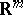.

In a mathematical system with given coordinates, smaller systems are chosen by equations or inequalities expressing restrictions or "constraints" . If the non-degeneracy condition for a system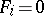is satisfied at all points of a manifold, then the gradients of the functions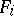form a framing or frame (a-frame orthogonal to the tangent plane at a point of the manifold and depending continuously on the point). Manifolds admitting a framing form the rather narrow class of stably-parallelizable manifolds (for example, they have orientations, cf. Orientation). But, locally, any differentiable manifold inmay be given by a non-degenerate system, and by using a partition of unity it is possible to construct a system of constant (but not maximal) rank which defines the manifold.

For a manifold given by an atlas, the problem arises of realizing it as a submanifold inwith regard to some-structure. Any topological, smooth or piecewise-linear manifoldis imbeddable, that is, is-homeomorphic to a submanifold in, and in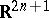the set of imbeddings is dense in the space of all continuous mappings. For other classes the question is significantly more complicated. It has been studied intensively, for example, for Riemannian manifolds. Algebraic varieties (cf. Algebraic variety), realized in a complex projective space (replacing here), constitute a very special class (Hodge varieties, cf. Hodge variety). If degenerate systems of equations are allowed, then manifolds with singularities arises. In algebraic geometry both ideas combine: An algebraic space is defined by joining pieces which are locally given by polynomial systems with degeneracies. In cobordism theory, in topology, under the name of manifold with singularities one discusses spaces which are constructed locally as products of cones over manifolds. Subsequent generalizations are connected with the consideration of continuous families of manifolds (see Fibre space; Fibration; Stratification).

The study of manifolds by pure topological means is very complicated and, until recently, was restricted to local properties, that is, virtually properties of(continuous decomposition; wild imbedding; etc.). The global study of manifolds necessarily requires the use of either homological or other means from algebraic topology, or smooth, piecewise-linear or other structures. The fundamental homological property of a manifold is Poincaré duality, which is also preserved for homology manifolds (cf. Homology manifold), and is used for generalizations of the notion of a manifold, such as Poincaré complexes. The degree of a mapping is homological in nature; this is a basic idea which is also defined for pseudo-manifolds, i.e. spaces (usually complexes) which have open connected dense subsets that are manifolds.

The geometric study of manifolds, based on the use of the various structures, comes first of all from the local equivalence of manifolds withand from the possibility, by approximating a mapping by smooth or piecewise-linear mappings, of putting them into general position, etc. The first problem here is the representation of a manifold as a space made up of simple pieces. The initial idea was that of triangulation, developing into the general idea of a complex. The difficult problems of triangulability and equivalence of triangulations were cleared up in the 1960's and 1970's (see Topology of manifolds). A more flexible tool has turned out to be handle decomposition of a manifold, equivalent to considering Morse functions. The basic theorems (on-cobordism, the generalized Poincaré conjecture, etc.) were proved using the technique of successive simplification of this decomposition. This technique also acts as a geometric foundation for the classification theorems on smooth and piecewise-linear structures in a given homotopy type of manifolds. These theorems, however, required the use of subtle invariants, connected with the tangent bundle of the manifold (see below). Of great significance was also the discovery of new homology theories:-theory and the theory of cobordism. Two manifolds are cobordant if together they bound a third manifold. Here the various structures are taken into account. This equivalence relation is based on the initial definition of homology, when cycles were considered naively as piecewise-smooth manifolds. In cobordism theory this idea was restored, but submanifolds were replaced by mappings of manifolds. The fundamental groups play a special role in questions on the classification of structures on manifolds, due to which the connection of these questions with algebraic-theory has been established.

The most important invariant of a smooth manifoldis its tangent bundle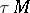. The subject of analysis on manifolds is the study of sections ofand the various bundles associated with it. From the topological point of viewis characterized by the existence of the exponential mapping, diffeomorphically mapping small balls in the fibres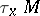onto neighbourhoods of the corresponding points. It can be described as a projection of a tubular neighbourhood of the diagonal in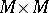onto a factor. In this form the definition ofcan be transferred to the topological, piecewise-linear, etc., cases (see Micro-bundle).

Different reductions of the structure group of the tangent bundle are called structures on the manifold. If an atlas can be chosen such that the Jacobi matrices of its coordinate transitions belong to a reduced structure group, and thus determine this reduction, then such a structure is called integrable. The question of classification of structures and their integrability is related to the fundamental questions of the differential geometry of manifolds. In the topology of manifolds the question on the classification of smooth, piecewise-linear and other structures reduces mainly to the classification of the corresponding structures on the tangent bundle and, by the same token, lead to homology problems.

The major role of the tangent bundle is connected with the fact that there are cohomology classes invariantly connected with it (cf. Characteristic class) in some homology theory, carrying the most essential information on the global structure of the manifold and connecting the properties of the different constructions and structures onwith its topological properties.

Research into the topology of manifolds in this direction, up to the 1970's, essentially used the smooth and piecewise-linear structures on manifolds (more precisely, the homotopy type of manifolds). The transition to pure topological results became possible only after the proof of difficult and deep theorems, beginning with the proof of the topological invariance of the (rational) characteristic classes (see Topology of manifolds). At the end of the 1970's this direction was merging with the above-mentioned pure topological study of manifolds. A vivid example is the proof of the conjecture that the dual suspension over a three-dimensional homology sphere is a manifold (a sphere). This allowed one to give a topological classification of manifolds (known up to that time only for one-dimensional and two-dimensional manifolds, cf. Two-dimensional manifold), to clarify the question of which polyhedra are manifolds (the only obstacle here is the, as yet unproved (1990), Poincaré conjecture in dimension 4) and others (see Topology of manifolds and ). The first period in the study of manifolds is related to the analysis

## Historical sketch

of the idea of a multi-dimensional parametrization, with research into the geometry of the physical world (the surface of the Earth) and into geometric axiomatics. The two methods for specifying a manifold in(local parametrization and equations) were first considered by C.F. Gauss (see , p. 127) for surfaces in, and in the multi-dimensional case by H. Poincaré (see ). J. Plücker  studied local coordinates in manifolds formed from curves, surfaces, etc. H. Grassmann arrived in  at the general idea of a "multi-dimensional extension" , which, under the name "manifold" , was introduced into mathematics by B. Riemann in his famous lecture Über die Hypothesen, welche der Geometrie zuGrunde liegen (see ). The properties of various special coordinates were studied by C.G.J. Jacobi, G. Lamé and others (see ).

Gauss (see , p. 123), in connection with his own work on geodesy, began a systematic study of surfaces, introducing the notion of intrinsic geometry, and thus that of manifold, not depending on the ambient number space, and in fact the notion of a structure on a manifold. This idea was completely understood only in the theory of characteristic classes, constructed in the mid 20th century. Riemann translated the ideas of Gauss to multi-dimensional manifolds. On the basis of Riemannian geometry, tensor analysis was created by G. Ricci, T. Levi-Civita, E.B. Christoffel, and others, the further development of which went in close connection with relativity theory. Another geometric line of development of the notion of a manifold was made initially in the discovery of the possibility of non-Euclidean geometries, and in the construction of geometry on the basis of the notion of a motion (H. Helmholtz, see , p. 366). This idea was transformed in a general program of group-theoretic constructions of geometry by F. Klein (see , p. 399 and ) and led to the sophisticated work of S. Lie on the theory of continuous groups. The line of Helmholtz–Klein–Lie remained for a long time apart from the line of Gauss–Riemann–Ricci. It borrowed the notion of curvature from the latter, but was interested only in Klein spaces (cf. Klein space). However, important questions arose in the global structure of Lie groups and their homogeneous spaces and this attracted the attention to the global structure of manifolds. An important fact, producing a deep impression, was the discovery by Klein of elliptic geometry, locally equivalent to spherical geometry, but globally having essentially different properties, and also the discovery by A.F. Möbius and Klein of the phenomenon of non-orientability.

The synthesis of both directions took place in the papers of E. Cartan (see , p. 483). Starting from the research of G. Darboux on the theory of surfaces, he considered the moving-frame method for arbitrary manifolds inand came to the theory of structure equations, a distant relative of Darboux's theory including the work of Lie. In Cartan's method the notion of a-structure unified the ideas of Riemannian geometry and the theory of Lie groups. Essentially, Cartan introduced the notion of a tangent bundle and its structure group, which finally took shape only in the 1940's (see ). This idea also allowed a unification of mathematical analysis on manifolds with the topological study of manifolds. The foundation was provided by the de Rham isomorphism (see de Rham theorem) — the final form of Poincaré's idea (see ) on the connection between real cohomology and differential forms. The most important subsequent stage was the introduction of characteristic classes and their expression as integrals of forms, expressed in terms of the curvature form (an example here is the expression of the Euler characteristic in the Gauss–Bonnet theorem, originally due to W. van Dyck, see ).

The topological study of manifolds was initiated with the discovery of Riemann surfaces (cf. Riemann surface) in connection with the representation of complex-analytic functions as integrals, as an attempt to free oneself from the multivalency of these functions. The "periods" of integrals led to the notion of a connectivity number and, in the end, to homology. The notion of a multi-dimensional generalization of this idea and the idea of a global homological study of manifolds is due to Riemann (see ). This study was begun by Poincaré, who made a number of important discoveries and proved Poincaré duality. A stimulating role, following Riemann's discovery, was played by the study of two-dimensional manifolds (in the first place by Möbius and C. Jordan, see ), leading to their complete classification. Finally, it proved possible to progress only after clarifying the idea of a "pure" homeomorphism (Poincaré, for example, essentially used piecewise-smooth homeomorphisms). This clarification was one of the results of the analysis of the numerical continuum, undertoken at the end of the 19th century. Of great significance in this direction was the posing of Hilbert's fifth problem and the work of L.E.J. Brouwer , who proved theorems (on invariance of domain and invariance of dimension) which permitted H. Weyl  to formulate the notion of a topological manifold. However, in higher dimensions the topological study of manifolds for a long time was conducted within the limits of smooth and piecewise-linear structures. Smooth structures, introduced in , were mainly analyzed by H. Whitney , and also by G. Whitehead and others. Piecewise-linear structures were introduced by Brouwer and analyzed by J.W. Alexander  and also by M. Newman and Whitehead. For a long time they were considered only as an auxiliary means for the topological study of manifolds. Only in the 1950's the non-uniqueness of differentiable structures, even on spheres, was discovered, and at the end of the 1960's the possibility of non-uniqueness of piecewise-linear structures (for example, on tori) was discovered. Since the 1950's the study of manifolds has moved to the synthesis of ideas of topology and analysis, based in the first place on the idea of characteristic classes (see ).